← Back to homepage

# How to use TensorBoard with PyTorch

## November 10, 2021 by Chris

When your Deep Learning model is training, you are interested in how well it performs. Often, it's possible to output a variety of metrics on the fly. But did you know that there are tools for visualizing how performance evolves over time - and even allowing you to see performance over time after training was finished?

TensorBoard is one such tool. Originally intended for the TensorFlow library (including Keras models), it's a web application which reads log files from a directory and displays a variety of charts that can be very useful. Fun fact: it's also available for PyTorch! And precisely that is what we're going to build in today's article.

First of all, we're going to start with taking a look at TensorBoard. What is it capable of doing? How is TensorBoard available in PyTorch (hint: through the SummaryWriter)? This includes working on a real example that adds TensorBoard to your PyTorch model.

Are you ready? Let's take a look! 😎

## What is TensorBoard?

People who create stuff can usually know best how to describe what they created - and the same is true for the creators of TensorBoard:

In machine learning, to improve something you often need to be able to measure it. TensorBoard is a tool for providing the measurements and visualizations needed during the machine learning workflow. It enables tracking experiment metrics like loss and accuracy, visualizing the model graph, projecting embeddings to a lower dimensional space, and much more.

TensorBoard – Get Started

In other words, it's a tool for visualizing the machine learning experiments you performed in a variety of ways.

Indeed, it's possible to generate a large amount of plots when using TensorBoard. For example, using weight histograms, it's possible to see how the distribution of your layer weights evolved over time - in this case, over five epochs: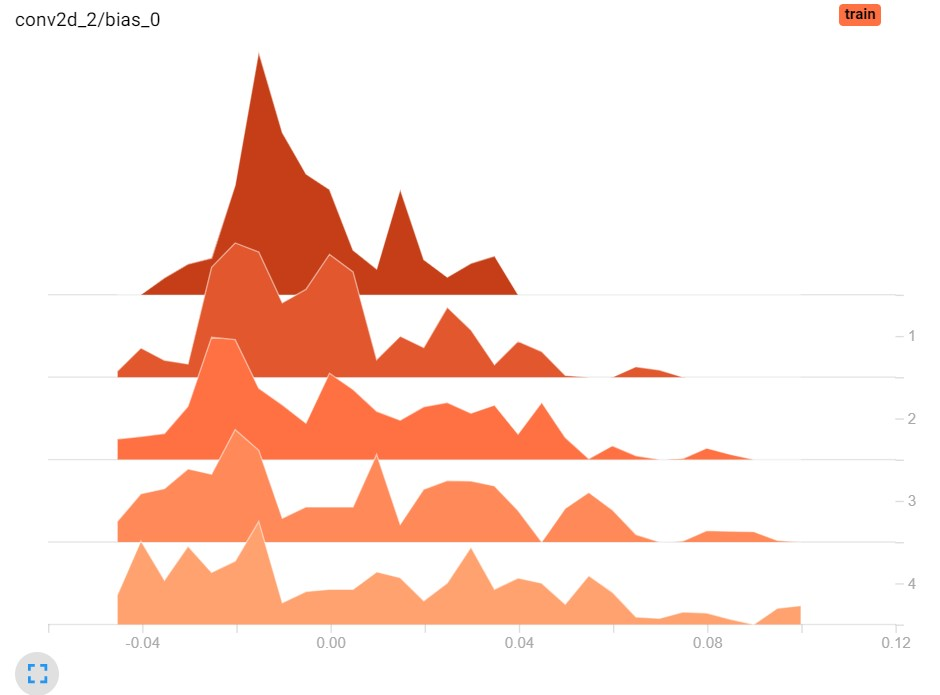In another screen, you can see how loss has evolved over the epochs: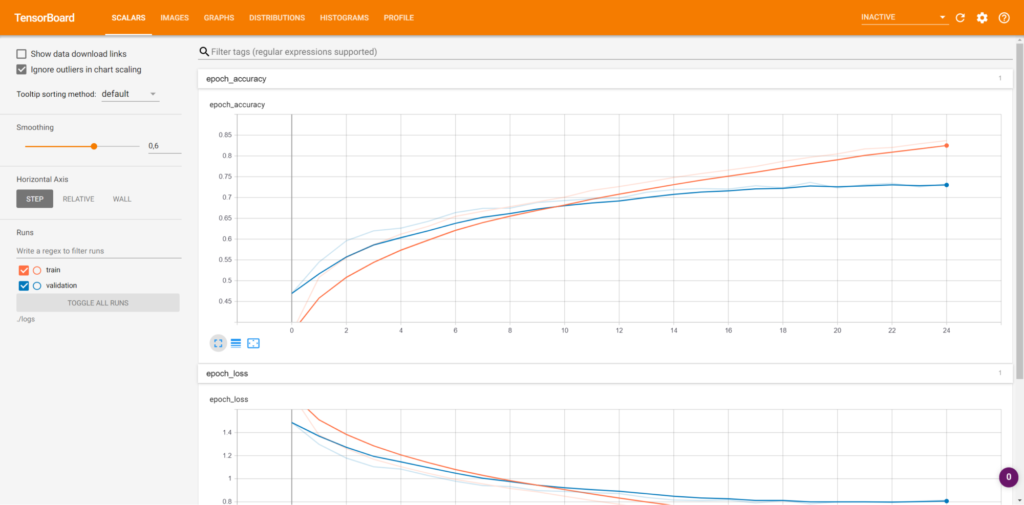And so on. And so on.

Installing TensorBoard must be done separately to your PyTorch install. Doing so is not difficult, fortunately, and can be done by simply executing pip via pip install tensorboard.

## TensorBoard in PyTorch using the SummaryWriter

TensorBoard was originally developed for TensorFlow. As you saw above, it is also available for PyTorch! But how? Through the SummaryWriter:

The SummaryWriter class provides a high-level API to create an event file in a given directory and add summaries and events to it. The class updates the file contents asynchronously. This allows a training program to call methods to add data to the file directly from the training loop, without slowing down training.

PyTorch (n.d.)

Great!

This means that we can create a SummaryWriter (or, fully: torch.utils.tensorboard.writer.SummaryWriter) and use it to write away the data that we want.

Recall from the article linked above that TensorBoard provides a variety of tabs:

• The scalar tab for showing how the training process happened over time by means of displaying scalars (e.g., in a line plot).
• The images tab for showing images written away during the training process.
• The graphs tab showing the network graph created by (in the original case) TensorFlow during training.
• The distributions tab showing the distributions of the weights and biases of your network for every iteration.
• The histograms tab showing the weight and bias histograms helping you determine how the model learned what it learned.
• The embeddings tab visualizes learned embeddings.

It's possible to write from PyTorch to each of these tabs:

• Using add_scalar (or add_scalars), you can write scalar data to the scalar tab.
• By means of add_image (or add_images), images can be written to the images tab. Besides, it is also possible to write Matplotlib figures using add_figure. And if you have videos (for example by having an array with multiple images), add_video can be used.
• Through add_graph, graph data can be written to TensorBoard's graphs tab.
• With add_histogram, you can write histogram data to the histogram tab.
• Via add_embedding, embedding data can be written to the embeddings tab.
• You can also use add_audio for audio data and add_text for text data. add_mesh can be used for 3D point cloud data.
• And there is a lot more!

In other words, it's possible to fully recreate the TensorBoard experience you're used to when coming from TensorFlow... but then using PyTorch!

Let's now take a look at how we can use TensorBoard with PyTorch by means of an example.

Please ensure that you have installed both PyTorch (and its related packages such as torchvision) as well as TensorBoard (if not: pip install tensorboard) before continuing.

Adding TensorBoard to your PyTorch model will take a few simple steps:

1. Starting with a simple Convolutional Neural Network.
2. Initializing the SummaryWriter which allows us to write to TensorBoard.
3. Writing away some scalar values, both individually and in groups.
4. Writing away images, graphs and histograms.

This will give you a rough idea how TensorBoard can be used, leaving sufficient room for experimentation with all the other TensorBoard functionality available in PyTorch.

In a different article, we created a simple Convolutional Neural Network for classifying MNIST digits. Let's use that code here and expand it with the SummaryWriter for

import os
import torch
from torch import nn
from torchvision.datasets import MNIST
from torchvision import transforms

class ConvNet(nn.Module):
'''
Simple Convolutional Neural Network
'''
def __init__(self):
super().__init__()
self.layers = nn.Sequential(
nn.Conv2d(1, 10, kernel_size=3),
nn.ReLU(),
nn.Conv2d(10, 5, kernel_size=3),
nn.ReLU(),
nn.Flatten(),
nn.Linear(24 * 24 * 5, 64),
nn.ReLU(),
nn.Linear(64, 32),
nn.ReLU(),
nn.Linear(32, 10)
)

def forward(self, x):
'''Forward pass'''
return self.layers(x)

if __name__ == '__main__':

# Set fixed random number seed
torch.manual_seed(42)

# Prepare CIFAR-10 dataset

# Initialize the ConvNet
convnet = ConvNet()

# Define the loss function and optimizer
loss_function = nn.CrossEntropyLoss()

# Run the training loop
for epoch in range(0, 5): # 5 epochs at maximum

# Print epoch
print(f'Starting epoch {epoch+1}')

# Set current loss value
current_loss = 0.0

# Iterate over the DataLoader for training data
for i, data in enumerate(trainloader, 0):

# Get inputs
inputs, targets = data

# Perform forward pass
outputs = convnet(inputs)

# Compute loss
loss = loss_function(outputs, targets)

# Perform backward pass
loss.backward()

# Perform optimization
optimizer.step()

# Print statistics
current_loss += loss.item()
if i % 500 == 499:
print('Loss after mini-batch %5d: %.3f' %
(i + 1, current_loss / 500))
current_loss = 0.0

# Process is complete.
print('Training process has finished.')


### Initializing the SummaryWriter

Add the SummaryWriter to your imports first:

import os
import torch
from torch import nn
from torchvision.datasets import MNIST
from torch.utils.tensorboard import SummaryWriter
from torchvision import transforms


Then, directly after the __name__ check, initialize it:

if __name__ == '__main__':

# Initialize the SummaryWriter for TensorBoard
# Its output will be written to ./runs/
writer = SummaryWriter()


We can now use TensorBoard within PyTorch :)

### Writing scalar values and groups to TensorBoard from PyTorch

If we inspect the code above, a prime candidate for writing to TensorBoard is the loss value. It is a simple value and hence can be represented as a scalar, and thus be written using add_scalar.

First, we add a new counter just after we start the training loop:

  # Run the training loop
loss_idx_value = 0


Then, we add the add_scalar call to our code - we write away the current_loss variable for the current index value, which we then increase with one.

      # Print statistics
current_loss += loss.item()
loss_idx_value += 1
if i % 500 == 499:
print('Loss after mini-batch %5d: %.3f' %
(i + 1, current_loss / 500))
current_loss = 0.0


Because current_loss is reset after every 500th minibatch, we're likely going to see a wavy pattern.

Let's now run the Python script - and when training finishes, you can start TensorBoard as follows from the directory where your script is located:

tensorboard --logdir=runs


You should then see the following:

(pytorch) C:\Users\Chris\Test>tensorboard --logdir=runs
Serving TensorBoard on localhost; to expose to the network, use a proxy or pass --bind_all
TensorBoard 2.6.0 at http://localhost:6006/ (Press CTRL+C to quit)


Let's go visit localhost!

Indeed, a wavy pattern. This makes sense, because loss is reset continuously. Fortunately, we also see a lower loss range - indicating that our maximum loss per epoch is decreasing, suggesting that the model gets better.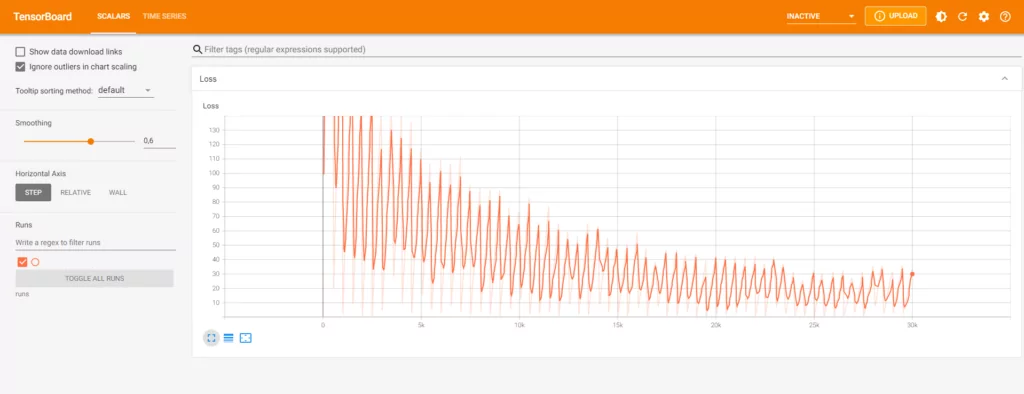If we want, we can also group multiple graphs in a scalar group, this way:

  # Run the training loop
loss_idx_value = 0
for epoch in range(0, 5): # 5 epochs at maximum

# Print epoch
print(f'Starting epoch {epoch+1}')

# Set current loss value
current_loss = 0.0

# Iterate over the DataLoader for training data
for i, data in enumerate(trainloader, 0):

# Get inputs
inputs, targets = data

# Perform forward pass
outputs = convnet(inputs)

# Compute loss
loss = loss_function(outputs, targets)

# Perform backward pass
loss.backward()

# Perform optimization
optimizer.step()

# Print statistics
current_loss += loss.item()
loss_idx_value += 1
if i % 500 == 499:
print('Loss after mini-batch %5d: %.3f' %
(i + 1, current_loss / 500))
current_loss = 0.0

# Write loss for epoch


We can now see loss at minibatch level (including the resets) and epoch level (which is more smooth) - all under the umbrella term of "Loss". This way, you can construct multiple groups which contain many visualizations.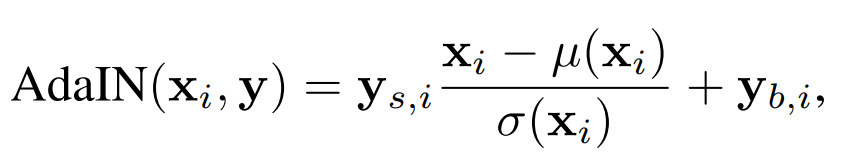### Writing network graphs, images and weight histograms to TensorBoard using PyTorch

#### Graphs

Using add_graph, you can write the network graph to TensorBoard so that it can be visualized. This is how to do it:

    # Iterate over the DataLoader for training data
for i, data in enumerate(trainloader, 0):

# Get inputs
inputs, targets = data

# Write the network graph at epoch 0, batch 0
if epoch == 0 and i == 0:


Et voila: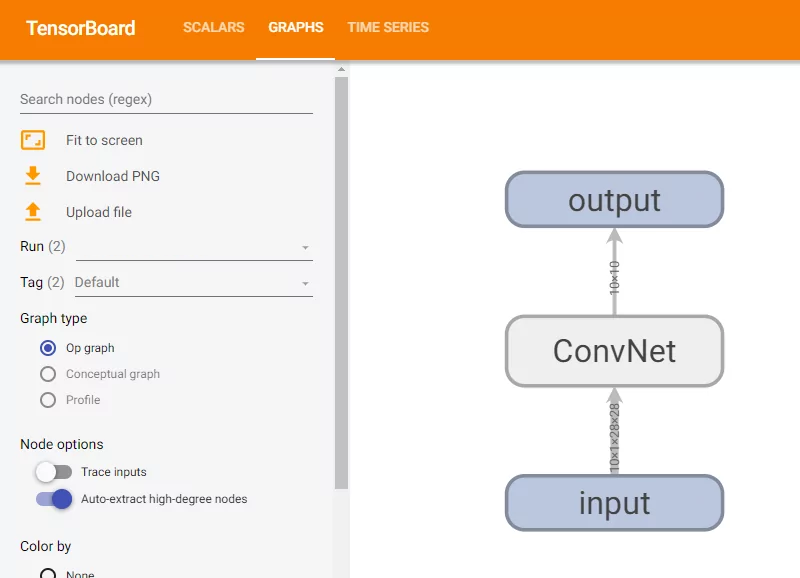#### Images

Suppose that you have image data available during the training process - for example, random selections from your batches, weight visualizations or e.g. Activation Maximization values - then you can use add_image in the following way:

      # Write an image at every batch 0
if i == 0:


At every 0th minibatch (i.e. at the start of every epoch), this writes the first input image to TensorBoard.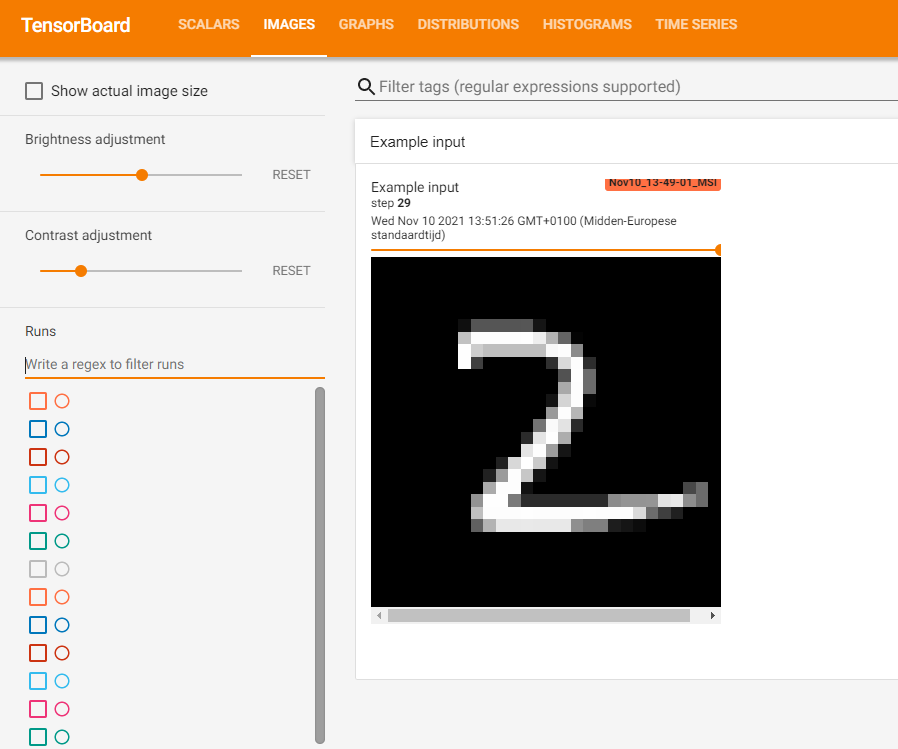#### Weight histograms

Visualizing weight histograms in TensorBoard takes a bit more time, because PyTorch doesn't natively support making weight histograms. Since its APIs are really accessible, it is not very difficult to replicate this behavior either. Let's take a look at what must be done for passing weight histograms to TensorBoard:

Please do note that the functionality below only works with Conv2d and Linear layers. All others will be skipped. If you need other layers, please feel free to let me know through the comments, and I will try to add them!

def weight_histograms_conv2d(writer, step, weights, layer_number):
weights_shape = weights.shape
num_kernels = weights_shape
for k in range(num_kernels):
flattened_weights = weights[k].flatten()
tag = f"layer_{layer_number}/kernel_{k}"

def weight_histograms_linear(writer, step, weights, layer_number):
flattened_weights = weights.flatten()
tag = f"layer_{layer_number}"

def weight_histograms(writer, step, model):
print("Visualizing model weights...")
# Iterate over all model layers
for layer_number in range(len(model.layers)):
# Get layer
layer = model.layers[layer_number]
# Compute weight histograms for appropriate layer
if isinstance(layer, nn.Conv2d):
weights = layer.weight
weight_histograms_conv2d(writer, step, weights, layer_number)
elif isinstance(layer, nn.Linear):
weights = layer.weight
weight_histograms_linear(writer, step, weights, layer_number)


Add these Python defs above the __main__ check. Here's what they do, from the bottom to the top one:

• With weight_histograms, you iterate over all layers in the model, check whether the layer is a nn.Conv2d or nn.Linear type of layer, and then proceed with the relevant definition.
• In weight_histograms_linear, we take the layer weights, flatten everything, and pass them to writer for making the histogram.
• In weight_histograms_conv2d, we do almost the same, except for doing it at kernel level. This is how it's done in TensorFlow too.

  # Run the training loop
loss_idx_value = 0
for epoch in range(0, 5): # 5 epochs at maximum

# Visualize weight histograms
weight_histograms(writer, epoch, convnet)


It's then possible to see weight histograms in your TensorBoard page (note that the network below was trained for 30 epochs):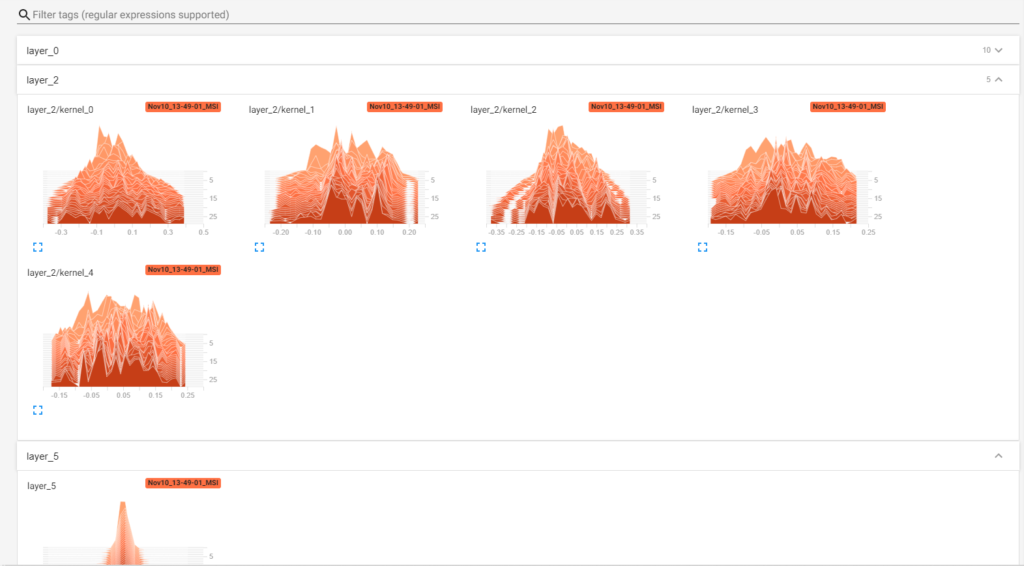## Full model code

Should you wish to use everything at once, here you go:

import os
import torch
from torch import nn
from torchvision.datasets import MNIST
from torch.utils.tensorboard import SummaryWriter
from torchvision import transforms
import numpy as np

class ConvNet(nn.Module):
'''
Simple Convolutional Neural Network
'''
def __init__(self):
super().__init__()
self.layers = nn.Sequential(
nn.Conv2d(1, 10, kernel_size=3),
nn.ReLU(),
nn.Conv2d(10, 5, kernel_size=3),
nn.ReLU(),
nn.Flatten(),
nn.Linear(24 * 24 * 5, 64),
nn.ReLU(),
nn.Linear(64, 32),
nn.ReLU(),
nn.Linear(32, 10)
)

def forward(self, x):
'''Forward pass'''
return self.layers(x)

def weight_histograms_conv2d(writer, step, weights, layer_number):
weights_shape = weights.shape
num_kernels = weights_shape
for k in range(num_kernels):
flattened_weights = weights[k].flatten()
tag = f"layer_{layer_number}/kernel_{k}"

def weight_histograms_linear(writer, step, weights, layer_number):
flattened_weights = weights.flatten()
tag = f"layer_{layer_number}"

def weight_histograms(writer, step, model):
print("Visualizing model weights...")
# Iterate over all model layers
for layer_number in range(len(model.layers)):
# Get layer
layer = model.layers[layer_number]
# Compute weight histograms for appropriate layer
if isinstance(layer, nn.Conv2d):
weights = layer.weight
weight_histograms_conv2d(writer, step, weights, layer_number)
elif isinstance(layer, nn.Linear):
weights = layer.weight
weight_histograms_linear(writer, step, weights, layer_number)

if __name__ == '__main__':

# Initialize the SummaryWriter for TensorBoard
# Its output will be written to ./runs/
writer = SummaryWriter()

# Set fixed random number seed
torch.manual_seed(42)

# Prepare CIFAR-10 dataset

# Initialize the ConvNet
convnet = ConvNet()

# Define the loss function and optimizer
loss_function = nn.CrossEntropyLoss()

# Run the training loop
loss_idx_value = 0
for epoch in range(0, 30): # 5 epochs at maximum

# Visualize weight histograms
weight_histograms(writer, epoch, convnet)

# Print epoch
print(f'Starting epoch {epoch+1}')

# Set current loss value
current_loss = 0.0

# Iterate over the DataLoader for training data
for i, data in enumerate(trainloader, 0):

if i > 1000:
break

# Get inputs
inputs, targets = data

# Write the network graph at epoch 0, batch 0
if epoch == 0 and i == 0:

# Write an image at every batch 0
if i == 0:

# Perform forward pass
outputs = convnet(inputs)

# Compute loss
loss = loss_function(outputs, targets)

# Perform backward pass
loss.backward()

# Perform optimization
optimizer.step()

# Print statistics
current_loss += loss.item()
loss_idx_value += 1
if i % 500 == 499:
print('Loss after mini-batch %5d: %.3f' %
(i + 1, current_loss / 500))
current_loss = 0.0

# Write loss for epoch

# Process is complete.
print('Training process has finished.')


That's it! You have successfully integrated your PyTorch model with TensorBoard.

## References

PyTorch. (n.d.). Torch.utils.tensorboard — PyTorch 1.7.0 documentationhttps://pytorch.org/docs/stable/tensorboard.html

TensorFlow. (n.d.). Get started with TensorBoardhttps://www.tensorflow.org/tensorboard/get_started